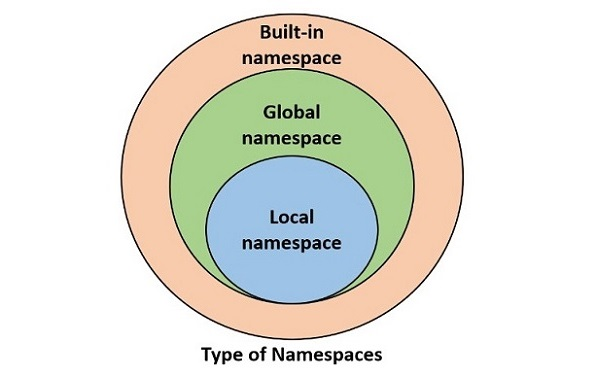# Python - Variable Scope

A variable in Python is a symbols name to the object in computer's memory. Python works on the concept of namespaces to define the context for various identifiers such as functions, variables etc. A namespace is a collection of symbolic names defined in the current context.

Python provides the following types of namespaces −

• Built-in namespace contains built-in functions and built-in exceptions. They are loaded in the memory as soon as Python interpreter is loaded and remain till the interpreter is running.

• Global namespace contains any names defined in the main program. These names remain in memory till the program is running.

• Local namespace contains names defined inside a function. They are available till the function is running.

These namespaces are nested one inside the other. Following diagram shows relationship between namespaces.The life of a certain variable is restricted to the namespace in which it is defined. As a result, it is not possible to access a variable present in the inner namespace from any outer namespace.

## globals() Function

Python's standard library includes a built-in function globals(). It returns a dictionary of symbols currently available in global namespace.

Run the globals() function directly from the Python prompt.

```>>> globals()
{'__name__': '__main__', '__doc__': None, '__package__': None, '__loader__': <class '_frozen_importlib.BuiltinImporter'>, '__spec__': None, '__annotations__': {}, '__builtins__': <module 'builtins' (built-in)>}
```

It can be seen that the builtins module which contains definitions of all built-in functions and built-in exceptions is loaded.

Save the following code that contains few variables and a function with few more variables inside it.

```name = 'TutorialsPoint'
marks = 50
result = True
def myfunction():
a = 10
b = 20
return a+b

print (globals())
```

Calling globals() from inside this script returns following dictionary object −

```{'__name__': '__main__', '__doc__': None, '__package__': None, '__loader__': <_frozen_importlib_external.SourceFileLoader object at 0x00000263E7255250>, '__spec__': None, '__annotations__': {}, '__builtins__': <module 'builtins' (built-in)>, '__file__': 'C:\\Users\\user\\examples\\main.py', '__cached__': None, 'name': 'TutorialsPoint', 'marks': 50, 'result': True, 'myfunction': <function myfunction at 0x00000263E72004A0>}
```

The global namespace now contains variables in the program and their values and the function object in it (and not the variables in the function).

## locals() Function

Python's standard library includes a built-in function locals(). It returns a dictionary of symbols currently available in namespace of the function.

Modify the above script to print dictionary of global and local namespaces from within the function.

```name = 'TutorialsPoint'
marks = 50
result = True
def myfunction():
a = 10
b = 20
c = a+b
print ("globals():", globals())
print ("locals():", locals())
return c
myfunction()
```

The output shows that locals() returns a dictionary of variables and their values currently available in the function.

```globals(): {'__name__': '__main__', '__doc__': None, '__package__': None, '__loader__': <_frozen_importlib_external.SourceFileLoader object at 0x00000169AE265250>, '__spec__': None, '__annotations__': {}, '__builtins__': <module 'builtins' (built-in)>, '__file__': 'C:\\Users\\mlath\\examples\\main.py', '__cached__': None, 'name': 'TutorialsPoint', 'marks': 50, 'result': True, 'myfunction': <function myfunction at 0x00000169AE2104A0>}
locals(): {'a': 10, 'b': 20, 'c': 30}
```

Since both globals() and locals functions return dictionary, you can access value of a variable from respective namespace with dictionary get() method or index operator.

```print (globals()['name']) #displays TutorialsPoint
print (locals().get('a')) #displays 10
```

## Namespace Conflict

If a variable of same name is present in global as well as local scope, Python interpreter gives priority to the one in local namespace.

```marks = 50 # this is a global variable
def myfunction():
marks = 70 # this is a local variable
print (marks)

myfunction()
print (marks) # prints global value
```

It will produce the following output

```70
50
```

If you try to manipulate value of a global variable from inside a function, Python raises UnboundLocalError.

```marks = 50 # this is a global variable
def myfunction():
marks = marks + 20
print (marks)

myfunction()
print (marks) # prints global value
```

It will produce the following output

```   marks = marks + 20
^^^^^
UnboundLocalError: cannot access local variable 'marks' where it is not associated with a value
```

To modify a global variable, you can either update it with a dictionary syntax, or use the global keyword to refer it before modifying.

```var1 = 50 # this is a global variable
var2 = 60 # this is a global variable
def myfunction():
"Change values of global variables"
globals()['var1'] = globals()['var1']+10
global var2
var2 = var2 + 20

myfunction()
print ("var1:",var1, "var2:",var2) #shows global variables with changed values
```

It will produce the following output

```var1: 60 var2: 80
```

Lastly, if you try to access a local variable in global scope, Python raises NameError as the variable in local scope can't be accessed outside it.

```var1 = 50 # this is a global variable
var2 = 60 # this is a global variable
def myfunction(x, y):
total = x+y
print ("Total is a local variable: ", total)

myfunction(var1, var2)
print (total) # This gives NameError
```

It will produce the following output −

```Total is a local variable: 110
Traceback (most recent call last):
File "C:\Users\user\examples\main.py", line 9, in <module>
print (total) # This gives NameError
^^^^^
NameError: name 'total' is not defined
```